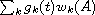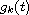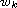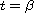Electron. J. Diff. Equ., Vol. 2014 (2014), No. 79, pp. 1-7.

### Explicit expressions for the matrix exponential function obtained by means of an algebraic convolution formula Jose Roberto Mandujano, Luis Verde-Star

Abstract:
We present a direct algebraic method for obtaining the matrix exponential function exp(tA), expressed as an explicit function of t for any square matrix A whose eigenvalues are known. The explicit form can be used to determine how a given eigenvalue affects the behavior of exp(tA). We use an algebraic convolution formula for basic exponential polynomials to obtain the dynamic solution g(t) associated with the characteristic (or minimal) polynomial w(x) of A. Then exp(tA) is expressed as, where theare successive derivatives of g and theare the Horner polynomials associated with w(x). Our method also gives a numberthat measures how well the computed approximation satisfies the matrix differential equation F'(tA)=A F(tA) at some given point. Some numerical experiments with random matrices indicate that the proposed method can be applied to matrices of order up to 40.

Submitted June 20, 2013. Published March 21, 2014.
Math Subject Classifications: 34A30, 65F60, 15A16.
Key Words: Matrix exponential; dynamic solutions; explicit formula; systems of linear differential equations.

Show me the PDF file (191 KB), TEX file, and other files for this article.

 José Roberto Mandujano Escuela Superior de Cómputo Instituto Politécnico Nacional U. Zacatenco, México D.F., México email: jrmandujano@yahoo.com.mx Luis Verde-Star Department of Mathematics Universidad Autónoma Metropolitana Iztapalapa, Apartado 55-534 México D.F. 09340, México email: verde@xanum.uam.mx